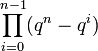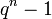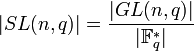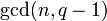# Order formulas for linear groups

This article gives a list of formulas for the orders of the general linear group of finite degree$n \ge 1$ and some other related groups, both for a finite field of size$q$ and for related rings.

## For a finite field of size$q$

### Formulas

In the formulas below, the field size is$q$ and the degree (order of matrices involved, dimension of vector space being acted upon) is$n$. The characteristic of the field is a prime number$p$.$q$ is a prime power with underlying prime$p$. We let$r = \log_pq$, so$q = p^r$ and$r$ is a nonnegative integer.

In the table below,$\Phi_d(q)$ stands for the$d^{th}$ cyclotomic polynomial evaluated at$q$.

Group Symbolic notation Order formula Order formula (powers of$q$ taken out) Order formula (maximally factorized) Degree as polynomial in$q$ (same as algebraic dimension) Multiplicity of factor$q$ Multiplicity of factor$q - 1$ Quick explanation for order
general linear group$GL(n,q)$ or$GL(n,\mathbb{F}_q)$$\prod_{i=0}^{n-1} (q^n - q^i)$$q^{\binom{n}{2}} \prod_{i=0}^{n-1} (q^{n-i} - 1)$$q^{\binom{n}{2}} \prod_{d=1}^n (\Phi_d(q))^{\lfloor n/d \rfloor}$$n^2$$\binom{n}{2} = \frac{n(n- 1)}{2}$$n$ See full explanation below.
special linear group$SL(n,q)$ or$SL(n,\mathbb{F}_q)$$\frac{\prod_{i=0}^{n-1} (q^n - q^i)}{q - 1}$$q^{\binom{n}{2}} \prod_{i=0}^{n-2} (q^{n-i} - 1)$$q^{\binom{n}{2}} (q - 1)^{n - 1} \prod_{d=2}^n (\Phi_d(q))^{\lfloor n/d \rfloor}$$n^2 - 1$$\binom{n}{2} = \frac{n(n- 1)}{2}$$n - 1$ See full explanation below.
projective general linear group$PGL(n,q)$ or$PGL(n,\mathbb{F}_q)$$\frac{\prod_{i=0}^{n-1} (q^n - q^i)}{q - 1}$$q^{\binom{n}{2}} \prod_{i=0}^{n-2} (q^{n-i} - 1)$$q^{\binom{n}{2}} (q - 1)^{n - 1} \prod_{d=2}^n (\Phi_d(q))^{\lfloor n/d \rfloor}$$n^2 - 1$$\binom{n}{2} = \frac{n(n- 1)}{2}$$n - 1$ See full explanation below.
projective special linear group$PSL(n,q)$ or$PSL(n,\mathbb{F}_q)$$\frac{\prod_{i=0}^{n-1} (q^n - q^i)}{(q - 1)\operatorname{gcd}(n,q-1)}$$q^{\binom{n}{2}} \frac{\prod_{i=0}^{n-2} (q^{n-i} - 1)}{\operatorname{gcd}(n,q-1)}$$q^{\binom{n}{2}} \frac{(q - 1)^{n - 1} \prod_{d=2}^n (\Phi_d(q))^{\lfloor n/d \rfloor}}{\operatorname{gcd}(n,q-1)}$$n^2 - 1$ (ignoring gcd term)$\binom{n}{2} = \frac{n(n- 1)}{2}$$n - 1$ (ignoring gcd term) See full explanation below.

### Explanation for order of general linear group

We describe here the reasoning behind the formula for the order of the general linear group$GL(n,q) = GL(n,\mathbb{F}_q)$.

The order equals the number of invertible$n \times n$ matrices with entries over$\mathbb{F}_q$. The set of uch matrices is in correpsondence with the set of ordered bases for$\mathbb{F}_q^n$, the$n$-dimensional vector space over$\mathbb{F}_q$ (for instance, we can identify the columns of the matrix with the vectors in the ordered basis). Thus, it suffices to count the number of possible ordered bases for$\mathbb{F}_q^n$.

For the first vector of the ordered basis, there are$q^n - 1$ possible choices (all nonzero vectors work). For the second vector, there are$q^n - q$ choices (all vectors that are not in the span of the first vector work). After the first$i$ basis vectors are chosen, the number of possibilities for the next basis vector are$q^n - q^i$, because$q^i$ is the size of the subspace spanned by the first$i$ basis vectors. By the product rule in combinatorics, we get that the total number of possibilities is:$\prod_{i=1}^n (q^n - q^i) = (q^n - 1)(q^n - q) \dots (q^n - q^{n-1})$

### Explanation for order of special linear group

The group$SL(n,q)$ is the kernel of the determinant map, a surjective homomorphism from$GL(n,q)$ to$\mathbb{F}_q^\ast$. The homomorphism is surjective because for any$a \in \mathbb{F}_q^\ast$, we can construct a diagonal matrix with one diagonal entry$a$ and the remaining entries equal to 1, and this maps to$a$ under the determinant map.

Essentially by Lagrange's theorem and the first isomorphism theorem, we know that the order of the kernel is the order of the whole group divided by the order of the image. Thus:$|SL(n,q)| = \frac{|GL(n,q)|}{|\mathbb{F}_q^\ast|}$

We now use the expression obtained for$|GL(n,q)|$ and use that$|\mathbb{F}_q^\ast| = q - 1$.

### Explanation for order of projective general linear group

The group$PGL(n,q)$ is the quotient of$GL(n,q)$ by the center of$GL(n,q)$.

The center of$GL(n,q)$ is the subgroup of scalar matrices (see center of general linear group is group of scalar matrices over center), and is isomorphic to$\mathbb{F}_q^\ast$.

Thus, by Lagrange's theorem, the order of the quotient$PGL(n,q)$ is:$|PGL(n,q)| = \frac{|GL(n,q)|}{|\mathbb{F}_q^\ast|}$

We now use the expression obtained for$|GL(n,q)|$ and use that$|\mathbb{F}_q^\ast| = q - 1$.

### Explanation for projective special linear group

The group$PSL(n,q)$ is the quotient group of$SL(n,q)$ by its intersection with the center of$GL(n,q)$.

The intersection of$SL(n,q)$ and the center of$GL(n,q)$ which is the subgroup of scalar matrices of determinant 1. The determinant of a$n \times n$ scalar matrix is the$n^{th}$ power of the scalar value, so the intersection comprises those scalar matrices whose scalar values are$n^{th}$ roots of unity. Since$\mathbb{F}_q^\ast$ is cyclic of order$q - 1$ (see multiplicative group of a finite field is cyclic), the number of such elements is$\operatorname{gcd}(n,q-1)$.

Thus, the kernel of the quotient map from$SL(n,q)$ to$PSL(n,q)$ has order$\operatorname{gcd}(n,q-1)$. We thus get, by Lagrange's theorem:$|PSL(n,q)| = \frac{|SL(n,q)|}{\operatorname{gcd}(n,q-1)}$

Simplifying this gives the expressions in the table above.